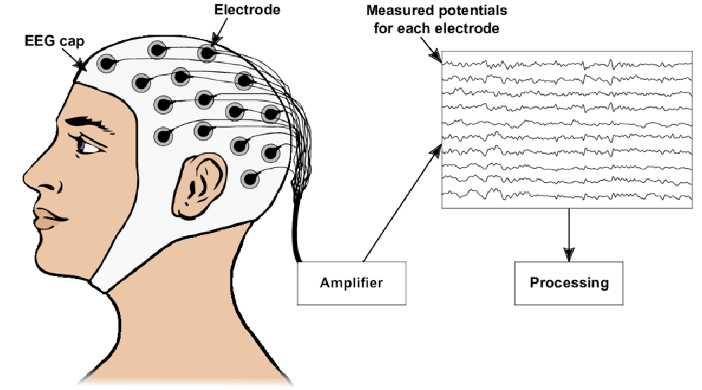# Online classification of covariance matrices for brain-computer interfaces and multimodal signalsFigure 1: Image credits: S. Nagel. “Towards a Home-Use BCI: Fast Asynchronous Control and Robust Non-Control State Detection”. Doctoral dissertation. Dec. 2019. (pdf)

## Description

A common approach in multimodal signal processing tasks (such as in EEG decoding) consists in representing several signals as a set of (dynamic) covariance matrices. For example, a temporal frame of an EEG recording, made of $$N$$ signals recorded over N electrodes (see the figure) can be represented as an $$N \times N$$ covariance matrix, each entry “$$ij$$” of the matrix corresponding to the cross-covariance of the recorded signals at electrodes “$$i$$” and “$$j$$” for that temporal frame. Representing such multimodal signals with time-varying covariance matrices allows us for instance to model the statistics of multimodal signals and to get rid of part of the noise affecting the raw signals.

In this context, the covariance matrices can be estimated either by batch processing, that is when all time instants are directly available for the estimation, or by online (or incremental) processing techniques where, at a given time, a previous estimate of a covariance matrix is updated according to new multimodal signal observations. This online processing is therefore closer to real-time applications (such as portable seizure detection from EEG sensors).

For this master project, we propose to work on algorithms for online covariance matrix processing when this matrix is assumed (approximately) low-rank; a common hypothesis that has been validated by previous studies.

## Objective

The objective of this processing will be either to detect abrupt change in the evolution of the covariance matrix (a change point detection problem); or to perform online classification of the covariance in a few labeled classes (such as those related to specific stimulus in an EEG recording).

The project will jointly consider two possible techniques for achieving these goals:

• data-driven deterministic methods estimating and updating the spectral properties of the covariance matrix (using singular value decomposition, shrinkage techniques, or subspace tracking);
• data-agnostic randomized methods (like rank-one projections) compressing the covariance matrices to a short feature vector representative of the spectral structure of that matrix; this technique is related to compressive sensing theory.

The designed algorithms will be applied to the EEG decoding problem by processing the SSVEP-based BCI recordings dataset.

This problem is of critical importance in the design of brain-computer interfaces, which are promising tools for helping physically-disabled people to communicate with their environment.

This master’s thesis will be co-supervised by Prof. Estelle Massart and Prof. Laurent Jacques (UCLouvain), with the help of Prof Pierre-Antoine Absil and Rémi Delogne (PhD student).

## References and possible research directions

• Incremental SVD and low-rank means for brain computer interfaces: Baker, C. G., Gallivan, K. A., & Van Dooren, P. (2012). Low-rank incremental methods for computing dominant singular subspaces. Linear Algebra and its Applications, 436(8), 2866-2888. (doi)
• Track the dominant subspace of the data: Zimmermann, R. (2021). A geometric approach to subspace updates and orthogonal matrix decompositions under rank-one modifications. Mathematics of Computation, 90(328), 671-688. (doi)
• Rank one projections:
• Cai, T. T., & Zhang, A. (2015). ROP: Matrix recovery via rank-one projections. The Annals of Statistics, 43(1), 102-138. (arXiv) + Video
• Chen, Y., Chi, Y., & Goldsmith, A. J. (2015). Exact and stable covariance estimation from quadratic sampling via convex programming. IEEE Transactions on Information Theory, 61(7), 4034-4059. (arXiv)
• Change-Point Detection: Keriven, N., Garreau, D., & Poli, I. (2020). NEWMA: a new method for scalable model-free online change-point detection. IEEE Transactions on Signal Processing, 68, 3515-3528.(arXiv)
• Brain computer interface and EEG: S. Chevallier et al, Review of Riemannian Distances and Divergences, Applied to SSVEP-based BCI, Neuroinformatics (2021) (HAL) Emmanuel K. Kalunga, Sylvain Chevallier, Quentin Barthelemy. Online SSVEP-based BCI using Riemannian Geometry. Neurocomputing, 2016 (arXiv).
• Dataset: SSVEP-based BCI recordings dataset https://github.com/sylvchev/dataset-ssvep-exoskeleton